SNAP Library 6.0, Developer Reference  2020-12-09 16:24:20 SNAP, a general purpose, high performance system for analysis and manipulation of large networks
TGHash< TDat > Class Template Reference

Graph Hash Table. More...

`#include <ghash.h>`

Collaboration diagram for TGHash< TDat >: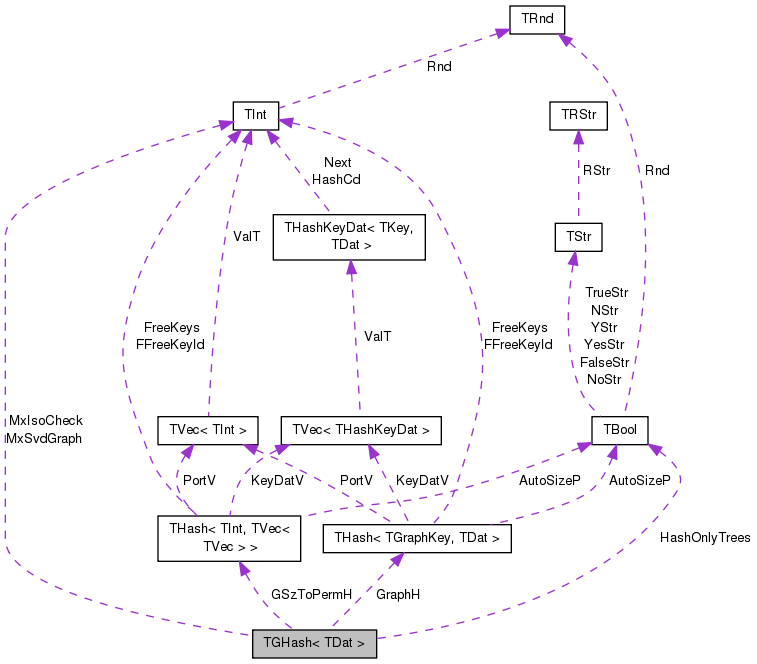[legend]

## Public Types

typedef THash< TGraphKey, TDat >::TIter TIter

## Public Member Functions

TGHash (const bool &HashTrees, const int &MaxIsoCheck=8, const int &MaxSvdGraph=500)
Default contructor. More...

TGHash (TSIn &SIn)

void Save (TSOut &SOut) const

const TDat & operator[] (const int &KeyId) const
Accesses the data at hash table position index KeyId. More...

TDat & operator[] (const int &KeyId)
Accesses the data at hash table position index KeyId. More...

const TDat & operator() (const TGraphKey &Key) const
Accesses the data of graph-key Key. More...

TDat & operator() (const TGraphKey &Key)
Accesses the data of graph-key Key. More...

TIter BegI () const
Returns iterator to the first element of the hash table. More...

TIter EndI () const
Returns iterator to one past the last element of the hash table. More...

TIter GetI (const int &KeyId) const
Returns iterator to a key at position index KeyId. More...

bool HashTrees () const
Returns whether the hash table only hashes trees and not arbitrary directed graphs. More...

void Gen (const int &ExpectVals)
Initializes the hash table for the expected number of keys ExpectVals. More...

void Clr (const bool &DoDel=true, const int &NoDelLim=-1)
Removes all the elements from the hash table. More...

bool Empty () const
Tests whether the hash table is empty. More...

int Len () const
Returns the number of keys in the hash table. More...

int GetPorts () const
Returns the number of ports in the hash table. More...

bool IsAutoSize () const
Tests whether the hash table automatically adjusts the number of ports based on the number of keys. More...

int GetMxKeyIds () const
Returns the maximum key Id of any element in the hash table. More...

bool IsKeyIdEqKeyN () const
Tests whether there are any unused slots in the hash table. More...

Adds a key Graph to the table and returns its KeyId. More...

TDat & AddDat (const PNGraph &Graph)
Adds a key Graph to the table and returns its data value. More...

TDat & AddDat (const PNGraph &Graph, const TDat &Dat)
Adds a key Graph to the table, sets its data value to value of Dat and returns Dat. More...

bool IsKey (const PNGraph &Graph) const
Test whether Graph is an existing key in the hash table. More...

int GetKeyId (const PNGraph &Graph) const
Returns the KeyId (position index) of key Graph. More...

const TDat & GetDat (const PNGraph &Graph) const
Returns the data associated with key Graph. More...

TDat & GetDat (const PNGraph &Graph)
Returns the data associated with key Graph. More...

const TGraphKeyGetKey (const int &KeyId) const
Returns the GraphKey with position index KeyId. More...

int GetKeyId (const TGraphKey &Key) const
Returns the KeyId for a given Key. More...

bool IsKey (const TGraphKey &Key) const
Tests whether a given Key exists in the hash table. More...

bool IsKey (const TGraphKey &Key, int &KeyId) const
Tests whether a given Key exists in the hash table. More...

bool IsKeyId (const int &KeyId) const
Tests whether there exists a key at given position index KeyId. More...

const TDat & GetDat (const TGraphKey &Key) const
Returns data with a given graph Key. More...

TDat & GetDat (const TGraphKey &Key)
Returns data with a given graph Key. More...

const TDat & GetDatId (const int &KeyId) const
Returns data at a given position index KeyId. More...

TDat & GetDatId (const int &KeyId)
Returns data at a given position index KeyId. More...

void GetKeyDat (const int &KeyId, TGraphKey &Key, TDat &Dat) const
Returns Key and Data at a given position index KeyId. More...

bool IsKeyGetDat (const TGraphKey &Key, TDat &Dat) const
Test whether Key exists and sets its data to Dat. More...

bool GetNodeMap (const PNGraph &Graph, TIntPrV &NodeMapV) const
Returns the mapping of node Ids of the Graph to those of the graph-key in the hash table. More...

bool GetNodeMap (const PNGraph &Graph, TIntPrV &NodeMapV, int &KeyId) const
Returns the mapping of node Ids of the Graph to those of the graph-key in the hash table and the Graph KeyId. More...

int FFirstKeyId () const
Finds first KeyId. More...

bool FNextKeyId (int &KeyId) const
Finds next KeyId. More...

void GetKeyV (TVec< TGraphKey > &KeyV) const
Returns a vector of keys stored in the hash table. More...

void GetDatV (TVec< TDat > &DatV) const
Returns a vector of data elements stored in the hash table. More...

void GetKeyIdByDat (TIntV &KeyIdV, const bool &Asc=true) const
Returns a vector of KeyIds of hash table elements sorted by their data value. More...

void GetKeyIdByGSz (TIntV &KeyIdV, const bool &Asc=true) const
Returns a vector of KeyIds of hash table elements sorted by their graph size. More...

void GetKeyDatPrV (TVec< TPair< TGraphKey, TDat > > &KeyDatPrV) const
Returns a vector of pairs (Key, Data) elements stored in the hash table. More...

void GetDatKeyPrV (TVec< TPair< TDat, TGraphKey > > &DatKeyPrV) const
Returns a vector of pairs (Data, Key) elements stored in the hash table. More...

void Defrag ()
Removes unused slots from the hash table. More...

void Pack ()
Frees the unused memory by the hash table. More...

void DrawGViz (const int &KeyId, const TStr &OutFNmPref, const TStr &OutputType="gif", TStr Desc="") const
Saves a given graph with key Id KeyId in DOT format and calls the GraphViz to draw it. More...

void DrawGViz (const TIntV &KeyIdV, const TStr &OutFNmPref, const TStr &OutputType="gif") const
Saves a set of graphs with key Ids KeyIdV in DOT format and calls the GraphViz to draw them. More...

void SaveTxt (const TStr &OutFNm, const TStr &Desc, const TStr &DatColNm, const bool &SortByKeyVal=true) const
Saves all graphs stored in the hash table into a text file. More...

void SaveDetailTxt (const TStr &OutFNm, const TStr &Desc, const TStr &DatColNm) const
Saves all graphs stored in the hash table into a text file and include additional information. More...

## Private Member Functions

void InitPermutations ()

int IsGetKeyId (const PNGraph &Graph) const

int IsGetKeyId (const PNGraph &Graph, TGraphKey &GKey) const

int IsGetKeyId (const PNGraph &Graph, TGraphKey &GKey, TIntPrV &NodeMap) const

## Private Attributes

TInt MxIsoCheck

TInt MxSvdGraph

THash< TInt, TVec< TIntV > > GSzToPermH

TBool HashOnlyTrees

THash< TGraphKey, TDat > GraphH

## Detailed Description

### template<class TDat> class TGHash< TDat >

Graph Hash Table.

Keys in this hash table are (little) directed graphs. The class is useful for counting frequencies of small subgraphs or information cascades. For small graphs with less than MxIsoCheck nodes the class performs exact isomorphism checking. For graphs with less than MxSvdGraph nodes the class performs approximate isomorphism checking by comparing a numeric SVD-based signatures of two graphs. For graphs with more than MxSvdGraph nodes the class performs approximate isorphism checking by comparing only the signature based on simple graph statistics. For hashing trees (tree is encoded as a directed graph where children point to the parent) the class always performs exact isomorphism testing.

Definition at line 103 of file ghash.h.

## Member Typedef Documentation

template<class TDat>
 typedef THash::TIter TGHash< TDat >::TIter

Definition at line 105 of file ghash.h.

## Constructor & Destructor Documentation

template<class TDat >
 TGHash< TDat >::TGHash ( const bool & HashTrees, const int & MaxIsoCheck = `8`, const int & MaxSvdGraph = `500` )

Default contructor.

For hashing trees and not general graphs, set HashTrees=true. A tree is a directed graph where children point to the parent. In this case exact isomorphism checking will be performed. For hashing general graphs, set HashTrees=false. MaxIsoCheck is the maximum number of nodes for which we perform brute force exact isomorphism check. For larger graph the isomorphism test is only approximate.

Definition at line 304 of file ghash.h.

References TGHash< TDat >::InitPermutations().

304  :
305  MxIsoCheck(MaxIsoCheck), MxSvdGraph(MaxSvdGraph), GSzToPermH(), HashOnlyTrees(HashTrees), GraphH() {
306  if (! HashTrees) {
308  }
309 }
TBool HashOnlyTrees
Definition: ghash.h:110
THash< TInt, TVec< TIntV > > GSzToPermH
Definition: ghash.h:109
TInt MxIsoCheck
Definition: ghash.h:107
TInt MxSvdGraph
Definition: ghash.h:108
THash< TGraphKey, TDat > GraphH
Definition: ghash.h:111
bool HashTrees() const
Returns whether the hash table only hashes trees and not arbitrary directed graphs.
Definition: ghash.h:146
void InitPermutations()
Definition: ghash.h:287

Here is the call graph for this function: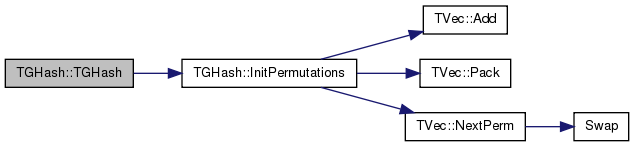template<class TDat >
 TGHash< TDat >::TGHash ( TSIn & SIn )

Definition at line 312 of file ghash.h.

References TGHash< TDat >::HashOnlyTrees, and TGHash< TDat >::InitPermutations().

312  : MxIsoCheck(SIn), MxSvdGraph(SIn), GSzToPermH(), HashOnlyTrees(SIn), GraphH(SIn) {
313  if (! HashOnlyTrees) {
315  }
316 }
TBool HashOnlyTrees
Definition: ghash.h:110
THash< TInt, TVec< TIntV > > GSzToPermH
Definition: ghash.h:109
TInt MxIsoCheck
Definition: ghash.h:107
TInt MxSvdGraph
Definition: ghash.h:108
THash< TGraphKey, TDat > GraphH
Definition: ghash.h:111
void InitPermutations()
Definition: ghash.h:287

Here is the call graph for this function:## Member Function Documentation

template<class TDat>
 TDat& TGHash< TDat >::AddDat ( const PNGraph & Graph )
inline

Adds a key Graph to the table and returns its data value.

If the key already exists the function returns the data associated with that existing key.

Definition at line 177 of file ghash.h.

Referenced by TDGHashGraphCounter::operator()().

THash< TGraphKey, TDat > GraphH
Definition: ghash.h:111
Adds a key Graph to the table and returns its KeyId.
Definition: ghash.h:327

Here is the caller graph for this function: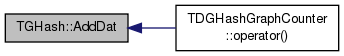template<class TDat>
 TDat& TGHash< TDat >::AddDat ( const PNGraph & Graph, const TDat & Dat )
inline

Adds a key Graph to the table, sets its data value to value of Dat and returns Dat.

If the key already exists the function sets the data value of that existing key.

Definition at line 181 of file ghash.h.

181 { return GraphH[AddKey(Graph)] = Dat; }
THash< TGraphKey, TDat > GraphH
Definition: ghash.h:111
Adds a key Graph to the table and returns its KeyId.
Definition: ghash.h:327
template<class TDat >
 int TGHash< TDat >::AddKey ( const PNGraph & Graph )

Adds a key Graph to the table and returns its KeyId.

KeyId is position index in the hash table. If the key already exists the function returns KeyId of that existing key.

Definition at line 327 of file ghash.h.

327  {
328  if (HashOnlyTrees) {
329  int RootNId; IAssert(TSnap::IsTree(Graph, RootNId));
330  TIntV TreeSig; TSnap::GetTreeSig(Graph, RootNId, TreeSig);
331  TGraphKey GKey(TreeSig);
332  const int KeyId = GraphH.GetKeyId(GKey);
333  if (KeyId == -1) {
334  GKey.TakeGraph(Graph);
336  }
337  return KeyId;
338  } else {
339  TGraphKey GKey;
340  GKey.TakeSig(Graph, MxIsoCheck+1, MxSvdGraph); // get signature
341  const int Nodes = GKey.GetNodes();
342  if (Nodes > 2 && Nodes <= MxIsoCheck) {
343  GKey.TakeGraph(Graph);
344  // Check all variants with same signature
345  for (int variant = 1; ; variant++) {
346  GKey.SetVariant(variant);
347  int KeyId = GraphH.GetKeyId(GKey);
348  if (KeyId == -1) { // Key of such signature and variant does not exist yet.
350  return KeyId;
351  }
352  if (TGraphKey::IsIsomorph(GKey, GraphH.GetKey(KeyId), GSzToPermH.GetDat(Nodes))) { // Graph isomorphism test
353  return KeyId; // Found isomorphic graph.
354  }
355  }
356  } else {
357  const int KeyId = GraphH.GetKeyId(GKey);
358  if (KeyId == -1) {
359  GKey.TakeGraph(Graph);
361  }
362  return KeyId;
363  }
364  }
365  Fail;
366  return -1;
367 }
void TakeSig(const PNGraph &Graph, const int &MnSvdGraph, const int &MxSvdGraph)
Creates a signature for a given directed graph.
Definition: ghash.cpp:94
#define IAssert(Cond)
Definition: bd.h:262
TBool HashOnlyTrees
Definition: ghash.h:110
#define Fail
Definition: bd.h:238
THash< TInt, TVec< TIntV > > GSzToPermH
Definition: ghash.h:109
static bool IsIsomorph(const TGraphKey &Key1, const TGraphKey &Key2, const TIntV &NodeIdMap)
Checks whether directed graph Key1 is isomorphic to the directed graph Key2 under node Id permutation...
Definition: ghash.cpp:186
void GetTreeSig(const PGraph &Graph, const int &RootNId, TIntV &Sig)
Definition: alg.h:484
bool IsTree(const PGraph &Graph, int &RootNIdX)
Definition: alg.h:460
TInt MxIsoCheck
Definition: ghash.h:107
TInt MxSvdGraph
Definition: ghash.h:108
void TakeGraph(const PNGraph &Graph)
Creates a key from a given directed graph.
Definition: ghash.cpp:58
int GetNodes() const
Returns the number of nodes in the graph.
Definition: ghash.h:31
int GetKeyId(const TKey &Key) const
Definition: hash.h:466
Definition: hash.h:373
Small Directed Graphs.
Definition: ghash.h:7
THash< TGraphKey, TDat > GraphH
Definition: ghash.h:111
void SetVariant(const int &Variant)
Sets the Variant Id of a given graph.
Definition: ghash.h:47
const TKey & GetKey(const int &KeyId) const
Definition: hash.h:252

Here is the call graph for this function: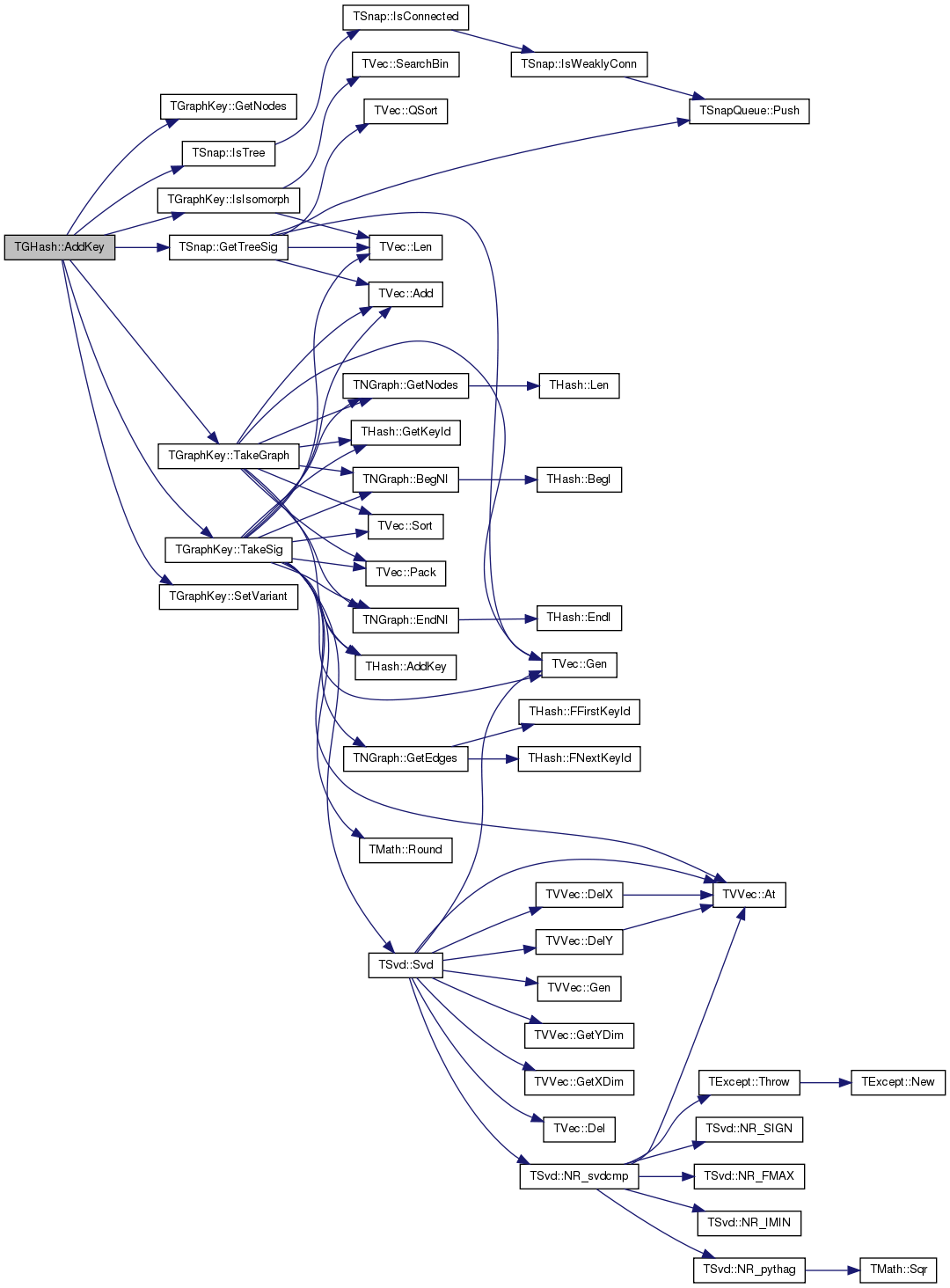Here is the caller graph for this function: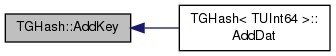template<class TDat>
 TIter TGHash< TDat >::BegI ( ) const
inline

Returns iterator to the first element of the hash table.

Definition at line 139 of file ghash.h.

139 { return GraphH.BegI(); }
TIter BegI() const
Definition: hash.h:213
THash< TGraphKey, TDat > GraphH
Definition: ghash.h:111
template<class TDat>
 void TGHash< TDat >::Clr ( const bool & DoDel = `true`, const int & NoDelLim = `-1` )
inline

Removes all the elements from the hash table.

If DoDel=true the data structure will free the memory, otherwise the object stays initialized (which makes subsequent insertion of keys much faster).

Definition at line 154 of file ghash.h.

154 { GraphH.Clr(DoDel, NoDelLim); }
THash< TGraphKey, TDat > GraphH
Definition: ghash.h:111
void Clr(const bool &DoDel=true, const int &NoDelLim=-1, const bool &ResetDat=true)
Definition: hash.h:361
template<class TDat>
 void TGHash< TDat >::Defrag ( )
inline

Removes unused slots from the hash table.

Slots get freed after removing keys from the table.

Definition at line 272 of file ghash.h.

272 { GraphH.Defrag(); }
void Defrag()
Definition: hash.h:555
THash< TGraphKey, TDat > GraphH
Definition: ghash.h:111
template<class TDat >
 void TGHash< TDat >::DrawGViz ( const int & KeyId, const TStr & OutFNmPref, const TStr & OutputType = `"gif"`, TStr Desc = `""` ) const

Saves a given graph with key Id KeyId in DOT format and calls the GraphViz to draw it.

Definition at line 480 of file ghash.h.

480  {
481  IAssert(OutputType == "ps" || OutputType == "gif" || OutputType == "png");
482  const TGraphKey& GKey = GetKey(KeyId);
483  const TStr Desc1 = TStr::Fmt("%s (%d, %d)", Desc.CStr(), GKey.GetNodes(), GKey.GetEdges());
484  GKey.SaveGViz(OutFNmPref+".dot", Desc1);
485  TSnap::TSnapDetail::GVizDoLayout(OutFNmPref+".dot", OutFNmPref+"."+OutputType, gvlDot);
486 }
int GetEdges() const
Returns the number of edges in the graph.
Definition: ghash.h:33
#define IAssert(Cond)
Definition: bd.h:262
const TGraphKey & GetKey(const int &KeyId) const
Returns the GraphKey with position index KeyId.
Definition: ghash.h:199
void GVizDoLayout(const TStr &GraphInFNm, TStr OutFNm, const TGVizLayout &Layout)
Runs GraphViz layout engine over a graph saved in the file GraphInFNm with output saved to OutFNm...
Definition: gviz.cpp:5
Definition: gviz.h:3
int GetNodes() const
Returns the number of nodes in the graph.
Definition: ghash.h:31
void SaveGViz(const TStr &OutFNm, const TStr &Desc=TStr(), const TStr &NodeAttrs="", const int &Size=-1) const
Saves the graph to the .DOT file format used by GraphViz.
Definition: ghash.cpp:154
Small Directed Graphs.
Definition: ghash.h:7
Definition: dt.h:412
static TStr Fmt(const char *FmtStr,...)
Definition: dt.cpp:1599
char * CStr()
Definition: dt.h:479

Here is the call graph for this function: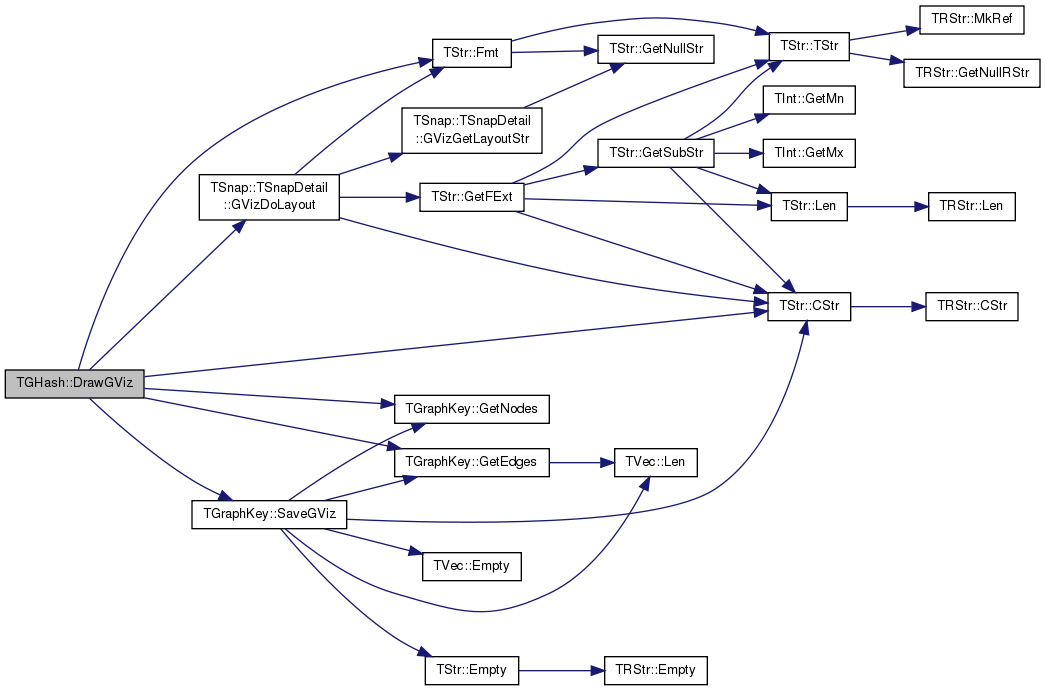template<class TDat >
 void TGHash< TDat >::DrawGViz ( const TIntV & KeyIdV, const TStr & OutFNmPref, const TStr & OutputType = `"gif"` ) const

Saves a set of graphs with key Ids KeyIdV in DOT format and calls the GraphViz to draw them.

Definition at line 489 of file ghash.h.

489  {
490  IAssert(OutputType == "ps" || OutputType == "gif" || OutputType == "png");
491  TExeTm ExeTm;
492  printf("Plotting %d graphs\n", KeyIdV.Len());
493  for (int i = 0; i < KeyIdV.Len(); i++) {
494  const TStr FNm = TStr::Fmt("%s.%03d.key%d.", OutFNmPref.CStr(), i+1, KeyIdV[i]());
495  const TStr Desc = TStr::Fmt("KeyId:%d", KeyIdV[i]());
496  const TGraphKey& GKey = GetKey(KeyIdV[i]);
497  printf("\r %d g(%d, %d) ", i, GKey.GetNodes(), GKey.GetEdges());
498  GKey.SaveGViz(FNm+"dot", Desc);
499  TSnap::TSnapDetail::GVizDoLayout(FNm+"dot", FNm+OutputType, gvlDot);
500  }
501  printf("done [%s].\n", ExeTm.GetTmStr());
502 }
int GetEdges() const
Returns the number of edges in the graph.
Definition: ghash.h:33
#define IAssert(Cond)
Definition: bd.h:262
const TGraphKey & GetKey(const int &KeyId) const
Returns the GraphKey with position index KeyId.
Definition: ghash.h:199
Definition: tm.h:355
TSizeTy Len() const
Returns the number of elements in the vector.
Definition: ds.h:575
const char * GetTmStr() const
Definition: tm.h:370
void GVizDoLayout(const TStr &GraphInFNm, TStr OutFNm, const TGVizLayout &Layout)
Runs GraphViz layout engine over a graph saved in the file GraphInFNm with output saved to OutFNm...
Definition: gviz.cpp:5
Definition: gviz.h:3
int GetNodes() const
Returns the number of nodes in the graph.
Definition: ghash.h:31
void SaveGViz(const TStr &OutFNm, const TStr &Desc=TStr(), const TStr &NodeAttrs="", const int &Size=-1) const
Saves the graph to the .DOT file format used by GraphViz.
Definition: ghash.cpp:154
Small Directed Graphs.
Definition: ghash.h:7
Definition: dt.h:412
static TStr Fmt(const char *FmtStr,...)
Definition: dt.cpp:1599
char * CStr()
Definition: dt.h:479

Here is the call graph for this function: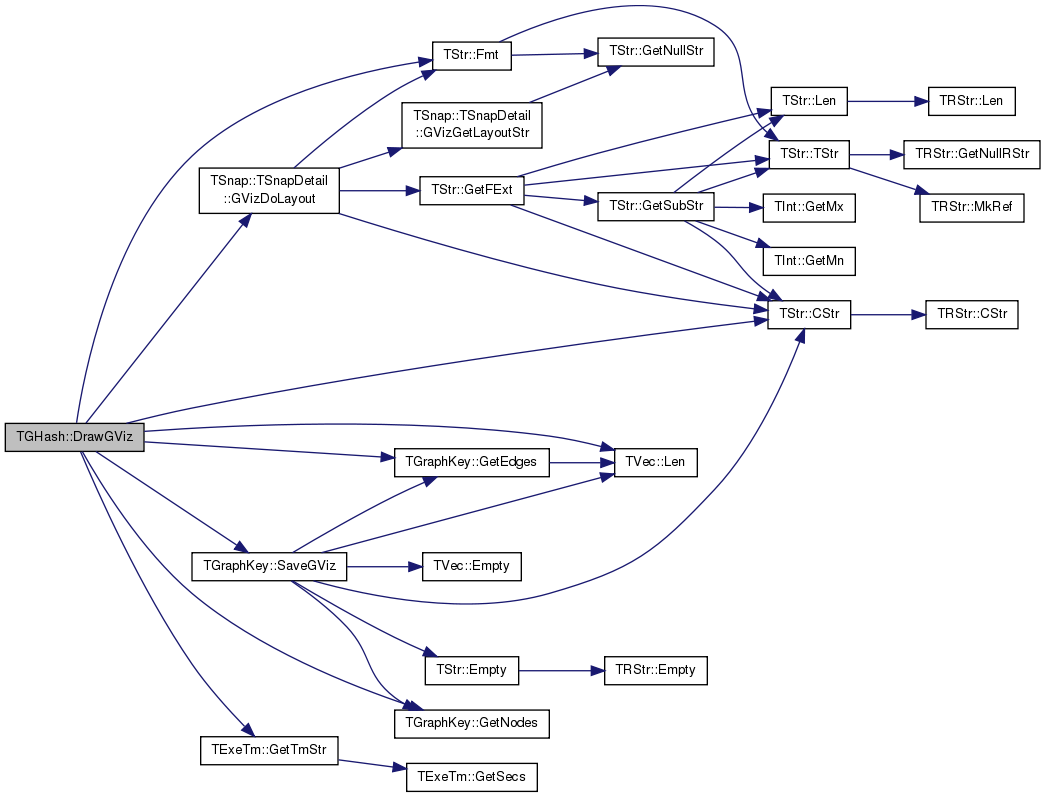template<class TDat>
 bool TGHash< TDat >::Empty ( ) const
inline

Tests whether the hash table is empty.

Definition at line 156 of file ghash.h.

156 { return GraphH.Empty(); }
bool Empty() const
Definition: hash.h:227
THash< TGraphKey, TDat > GraphH
Definition: ghash.h:111
template<class TDat>
 TIter TGHash< TDat >::EndI ( ) const
inline

Returns iterator to one past the last element of the hash table.

Definition at line 141 of file ghash.h.

141 { return GraphH.EndI(); }
TIter EndI() const
Definition: hash.h:218
THash< TGraphKey, TDat > GraphH
Definition: ghash.h:111
template<class TDat>
 int TGHash< TDat >::FFirstKeyId ( ) const
inline

Finds first KeyId.

Used for traversing the elements of the hash table: for (int KeyId = GHash.FFirstKeyId(); GHash.FNextKeyId(KeyId); ) { }

Definition at line 245 of file ghash.h.

245 { return 0-1; }
template<class TDat>
 bool TGHash< TDat >::FNextKeyId ( int & KeyId ) const
inline

Finds next KeyId.

Used for traversing the elements of the hash table: for (int KeyId = GHash.FFirstKeyId(); GHash.FNextKeyId(KeyId); ) { }

Definition at line 250 of file ghash.h.

250 { return GraphH.FNextKeyId(KeyId); }
bool FNextKeyId(int &KeyId) const
Definition: hash.h:478
THash< TGraphKey, TDat > GraphH
Definition: ghash.h:111
template<class TDat>
 void TGHash< TDat >::Gen ( const int & ExpectVals )
inline

Initializes the hash table for the expected number of keys ExpectVals.

Definition at line 149 of file ghash.h.

149 { GraphH.Gen(ExpectVals); }
void Gen(const int &ExpectVals)
Definition: hash.h:222
THash< TGraphKey, TDat > GraphH
Definition: ghash.h:111
template<class TDat>
 const TDat& TGHash< TDat >::GetDat ( const PNGraph & Graph ) const
inline

Returns the data associated with key Graph.

If the key does not exist the function aborts.

Definition at line 192 of file ghash.h.

Referenced by TDGHashGraphCounter::operator()().

192 { return GraphH[GetKeyId(Graph)]; }
int GetKeyId(const PNGraph &Graph) const
Returns the KeyId (position index) of key Graph.
Definition: ghash.h:188
THash< TGraphKey, TDat > GraphH
Definition: ghash.h:111

Here is the caller graph for this function: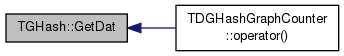template<class TDat>
 TDat& TGHash< TDat >::GetDat ( const PNGraph & Graph )
inline

Returns the data associated with key Graph.

If the key does not exist the function aborts.

Definition at line 196 of file ghash.h.

196 { return GraphH[GetKeyId(Graph)]; }
int GetKeyId(const PNGraph &Graph) const
Returns the KeyId (position index) of key Graph.
Definition: ghash.h:188
THash< TGraphKey, TDat > GraphH
Definition: ghash.h:111
template<class TDat>
 const TDat& TGHash< TDat >::GetDat ( const TGraphKey & Key ) const
inline

Returns data with a given graph Key.

If the key does not exist the function aborts.

Definition at line 213 of file ghash.h.

213 { return GraphH.GetDat(Key); }
const TDat & GetDat(const TKey &Key) const
Definition: hash.h:262
THash< TGraphKey, TDat > GraphH
Definition: ghash.h:111
template<class TDat>
 TDat& TGHash< TDat >::GetDat ( const TGraphKey & Key )
inline

Returns data with a given graph Key.

If the key does not exist the function aborts.

Definition at line 217 of file ghash.h.

217 { return GraphH.GetDat(Key); }
const TDat & GetDat(const TKey &Key) const
Definition: hash.h:262
THash< TGraphKey, TDat > GraphH
Definition: ghash.h:111
template<class TDat>
 const TDat& TGHash< TDat >::GetDatId ( const int & KeyId ) const
inline

Returns data at a given position index KeyId.

If KeyId is out of bounds the function fails.

Definition at line 221 of file ghash.h.

221 { return GraphH[KeyId]; }
THash< TGraphKey, TDat > GraphH
Definition: ghash.h:111
template<class TDat>
 TDat& TGHash< TDat >::GetDatId ( const int & KeyId )
inline

Returns data at a given position index KeyId.

If KeyId is out of bounds the function fails.

Definition at line 225 of file ghash.h.

225 { return GraphH[KeyId]; }
THash< TGraphKey, TDat > GraphH
Definition: ghash.h:111
template<class TDat>
 void TGHash< TDat >::GetDatKeyPrV ( TVec< TPair< TDat, TGraphKey > > & DatKeyPrV ) const
inline

Returns a vector of pairs (Data, Key) elements stored in the hash table.

Definition at line 267 of file ghash.h.

267 { GraphH.GetDatKeyPrV(DatKeyPrV); }
void GetDatKeyPrV(TVec< TPair< TDat, TKey > > &DatKeyPrV) const
Definition: hash.h:511
THash< TGraphKey, TDat > GraphH
Definition: ghash.h:111
template<class TDat>
 void TGHash< TDat >::GetDatV ( TVec< TDat > & DatV ) const
inline

Returns a vector of data elements stored in the hash table.

Definition at line 254 of file ghash.h.

254 { GraphH.GetDatV(DatV); }
void GetDatV(TVec< TDat > &DatV) const
Definition: hash.h:492
THash< TGraphKey, TDat > GraphH
Definition: ghash.h:111
template<class TDat>
 TIter TGHash< TDat >::GetI ( const int & KeyId ) const
inline

Returns iterator to a key at position index KeyId.

Definition at line 143 of file ghash.h.

143 { return GraphH.GetI(KeyId); }
THash< TGraphKey, TDat > GraphH
Definition: ghash.h:111
TIter GetI(const TKey &Key) const
Definition: hash.h:220
template<class TDat>
 const TGraphKey& TGHash< TDat >::GetKey ( const int & KeyId ) const
inline

Returns the GraphKey with position index KeyId.

Definition at line 199 of file ghash.h.

199 { return GraphH.GetKey(KeyId); }
THash< TGraphKey, TDat > GraphH
Definition: ghash.h:111
const TKey & GetKey(const int &KeyId) const
Definition: hash.h:252
template<class TDat>
 void TGHash< TDat >::GetKeyDat ( const int & KeyId, TGraphKey & Key, TDat & Dat ) const
inline

Returns Key and Data at a given position index KeyId.

Definition at line 228 of file ghash.h.

228 { GraphH.GetKeyDat(KeyId, Key, Dat); }
void GetKeyDat(const int &KeyId, TKey &Key, TDat &Dat) const
Definition: hash.h:271
THash< TGraphKey, TDat > GraphH
Definition: ghash.h:111
template<class TDat>
 void TGHash< TDat >::GetKeyDatPrV ( TVec< TPair< TGraphKey, TDat > > & KeyDatPrV ) const
inline

Returns a vector of pairs (Key, Data) elements stored in the hash table.

Definition at line 265 of file ghash.h.

265 { GraphH.GetKeyDatPrV(KeyDatPrV); }
void GetKeyDatPrV(TVec< TPair< TKey, TDat > > &KeyDatPrV) const
Definition: hash.h:500
THash< TGraphKey, TDat > GraphH
Definition: ghash.h:111
template<class TDat>
 int TGHash< TDat >::GetKeyId ( const PNGraph & Graph ) const
inline

Returns the KeyId (position index) of key Graph.

If the key does not exist, the function returns -1.

Definition at line 188 of file ghash.h.

Referenced by TGHash< TUInt64 >::GetDat().

188 { return IsGetKeyId(Graph); }
int IsGetKeyId(const PNGraph &Graph) const
Definition: ghash.h:370

Here is the caller graph for this function: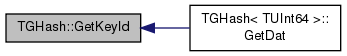template<class TDat>
 int TGHash< TDat >::GetKeyId ( const TGraphKey & Key ) const
inline

Returns the KeyId for a given Key.

If the key does not exist, the function returns -1.

Definition at line 203 of file ghash.h.

203 { return GraphH.GetKeyId(Key); }
int GetKeyId(const TKey &Key) const
Definition: hash.h:466
THash< TGraphKey, TDat > GraphH
Definition: ghash.h:111
template<class TDat >
 void TGHash< TDat >::GetKeyIdByDat ( TIntV & KeyIdV, const bool & Asc = `true` ) const

Returns a vector of KeyIds of hash table elements sorted by their data value.

Asc=true: sort in ascending order, otherwise in descending order.

Definition at line 454 of file ghash.h.

454  {
455  TVec<TQuad<TDat, TInt,TInt, TInt> > DatKeyIdV(Len(), 0); // <TDat,Nodes,Edges,KeyId>
456  for (int i = FFirstKeyId(); FNextKeyId(i); ) {
458  }
459  DatKeyIdV.Sort(Asc);
460  KeyIdV.Gen(Len(), 0);
461  for (int i = 0; i < Len(); i++) {
463  }
464 }
int Len() const
Returns the number of keys in the hash table.
Definition: ghash.h:158
const TGraphKey & GetKey(const int &KeyId) const
Returns the GraphKey with position index KeyId.
Definition: ghash.h:199
bool FNextKeyId(int &KeyId) const
Finds next KeyId.
Definition: ghash.h:250
const TDat & GetDatId(const int &KeyId) const
Returns data at a given position index KeyId.
Definition: ghash.h:221
int FFirstKeyId() const
Finds first KeyId.
Definition: ghash.h:245
Definition: ds.h:219
void Gen(const TSizeTy &_Vals)
Constructs a vector (an array) of _Vals elements.
Definition: ds.h:523
Adds a new element at the end of the vector, after its current last element.
Definition: ds.h:602
Vector is a sequence TVal objects representing an array that can change in size.
Definition: ds.h:430

Here is the call graph for this function: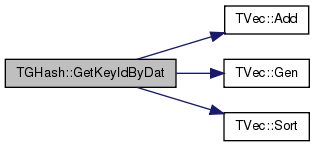template<class TDat >
 void TGHash< TDat >::GetKeyIdByGSz ( TIntV & KeyIdV, const bool & Asc = `true` ) const

Returns a vector of KeyIds of hash table elements sorted by their graph size.

Asc=true: sort in ascending order, otherwise in descending order. Graph size is the number of nodes and edges.

Definition at line 467 of file ghash.h.

467  {
468  TVec<TQuad<TInt,TInt, TDat, TInt> > DatKeyIdV(Len(), 0); // <Nodes,Edges,TDat,KeyId>
469  for (int i = FFirstKeyId(); FNextKeyId(i); ) {
471  }
472  DatKeyIdV.Sort(Asc);
473  KeyIdV.Gen(Len(), 0);
474  for (int i = 0; i < Len(); i++) {
476  }
477 }
int Len() const
Returns the number of keys in the hash table.
Definition: ghash.h:158
const TGraphKey & GetKey(const int &KeyId) const
Returns the GraphKey with position index KeyId.
Definition: ghash.h:199
bool FNextKeyId(int &KeyId) const
Finds next KeyId.
Definition: ghash.h:250
const TDat & GetDatId(const int &KeyId) const
Returns data at a given position index KeyId.
Definition: ghash.h:221
int FFirstKeyId() const
Finds first KeyId.
Definition: ghash.h:245
Definition: ds.h:219
void Gen(const TSizeTy &_Vals)
Constructs a vector (an array) of _Vals elements.
Definition: ds.h:523
Adds a new element at the end of the vector, after its current last element.
Definition: ds.h:602
Vector is a sequence TVal objects representing an array that can change in size.
Definition: ds.h:430

Here is the call graph for this function: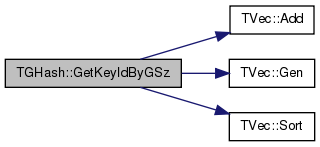template<class TDat>
 void TGHash< TDat >::GetKeyV ( TVec< TGraphKey > & KeyV ) const
inline

Returns a vector of keys stored in the hash table.

Definition at line 252 of file ghash.h.

252 { GraphH.GetKeyV(KeyV); }
void GetKeyV(TVec< TKey > &KeyV) const
Definition: hash.h:484
THash< TGraphKey, TDat > GraphH
Definition: ghash.h:111
template<class TDat>
 int TGHash< TDat >::GetMxKeyIds ( ) const
inline

Returns the maximum key Id of any element in the hash table.

Definition at line 164 of file ghash.h.

164 { return GraphH.GetMxKeyIds(); }
int GetMxKeyIds() const
Definition: hash.h:231
THash< TGraphKey, TDat > GraphH
Definition: ghash.h:111
template<class TDat >
 bool TGHash< TDat >::GetNodeMap ( const PNGraph & Graph, TIntPrV & NodeMapV ) const

Returns the mapping of node Ids of the Graph to those of the graph-key in the hash table.

The function assumes the Graph exists as a key in the table. Otherwise it returns false.

Definition at line 407 of file ghash.h.

407  {
408  int KeyId;
409  return GetNodeMap(Graph, NodeMapV, KeyId);
410 }
bool GetNodeMap(const PNGraph &Graph, TIntPrV &NodeMapV) const
Returns the mapping of node Ids of the Graph to those of the graph-key in the hash table...
Definition: ghash.h:407
template<class TDat >
 bool TGHash< TDat >::GetNodeMap ( const PNGraph & Graph, TIntPrV & NodeMapV, int & KeyId ) const

Returns the mapping of node Ids of the Graph to those of the graph-key in the hash table and the Graph KeyId.

The function assumes the Graph exists as a key in the table. Otherwise it returns false.

Definition at line 413 of file ghash.h.

413  {
414  NodeMapV.Clr(false);
415  if (HashOnlyTrees) {
416  int RootNId; IAssert(TSnap::IsTree(Graph, RootNId));
417  TIntV TreeSig; TSnap::GetTreeSig(Graph, RootNId, TreeSig, NodeMapV);
418  TGraphKey GKey(TreeSig);
419  KeyId = GraphH.GetKeyId(GKey);
420  return KeyId != -1;
421  } else {
422  const int Nodes = Graph->GetNodes();
423  int IsoPermId = -1;
424  NodeMapV.Clr(false);
425  if (Nodes == 0) { return true; }
426  else if (Nodes == 1) {
427  NodeMapV.Add(TIntPr(Graph->BegNI().GetId(), 0)); return true; }
428  else if (Nodes <= MxIsoCheck) {
429  TGraphKey GKey;
430  GKey.TakeSig(Graph, MxIsoCheck+1, MxSvdGraph);
431  GKey.TakeGraph(Graph, NodeMapV);
432  for (int variant = 1; ; variant++) {
433  GKey.SetVariant(variant);
434  KeyId = GraphH.GetKeyId(GKey);
435  if (KeyId == -1) { return false; }
436  if (TGraphKey::IsIsomorph(GKey, GraphH.GetKey(KeyId), GSzToPermH.GetDat(Nodes), IsoPermId)) {
437  const TIntV& K1K2Perm = GSzToPermH.GetDat(Nodes)[IsoPermId];
438  // map from graph to key1 to key2
439  for (int i = 0; i < NodeMapV.Len(); i++) {
440  NodeMapV[i].Val2 = K1K2Perm[NodeMapV[i].Val2]; }
441  return true;
442  }
443  }
444  return false;
445  } else {
446  return false; // graph too big to find the mapping
447  }
448  }
449  Fail;
450  return false;
451 }
void TakeSig(const PNGraph &Graph, const int &MnSvdGraph, const int &MxSvdGraph)
Creates a signature for a given directed graph.
Definition: ghash.cpp:94
#define IAssert(Cond)
Definition: bd.h:262
TPair< TInt, TInt > TIntPr
Definition: ds.h:83
TBool HashOnlyTrees
Definition: ghash.h:110
#define Fail
Definition: bd.h:238
TSizeTy Len() const
Returns the number of elements in the vector.
Definition: ds.h:575
THash< TInt, TVec< TIntV > > GSzToPermH
Definition: ghash.h:109
static bool IsIsomorph(const TGraphKey &Key1, const TGraphKey &Key2, const TIntV &NodeIdMap)
Checks whether directed graph Key1 is isomorphic to the directed graph Key2 under node Id permutation...
Definition: ghash.cpp:186
void GetTreeSig(const PGraph &Graph, const int &RootNId, TIntV &Sig)
Definition: alg.h:484
bool IsTree(const PGraph &Graph, int &RootNIdX)
Definition: alg.h:460
void Clr(const bool &DoDel=true, const TSizeTy &NoDelLim=-1)
Clears the contents of the vector.
Definition: ds.h:1022
TInt MxIsoCheck
Definition: ghash.h:107
TInt MxSvdGraph
Definition: ghash.h:108
void TakeGraph(const PNGraph &Graph)
Creates a key from a given directed graph.
Definition: ghash.cpp:58
int GetKeyId(const TKey &Key) const
Definition: hash.h:466
Small Directed Graphs.
Definition: ghash.h:7
THash< TGraphKey, TDat > GraphH
Definition: ghash.h:111
void SetVariant(const int &Variant)
Sets the Variant Id of a given graph.
Definition: ghash.h:47
Adds a new element at the end of the vector, after its current last element.
Definition: ds.h:602
const TKey & GetKey(const int &KeyId) const
Definition: hash.h:252

Here is the call graph for this function: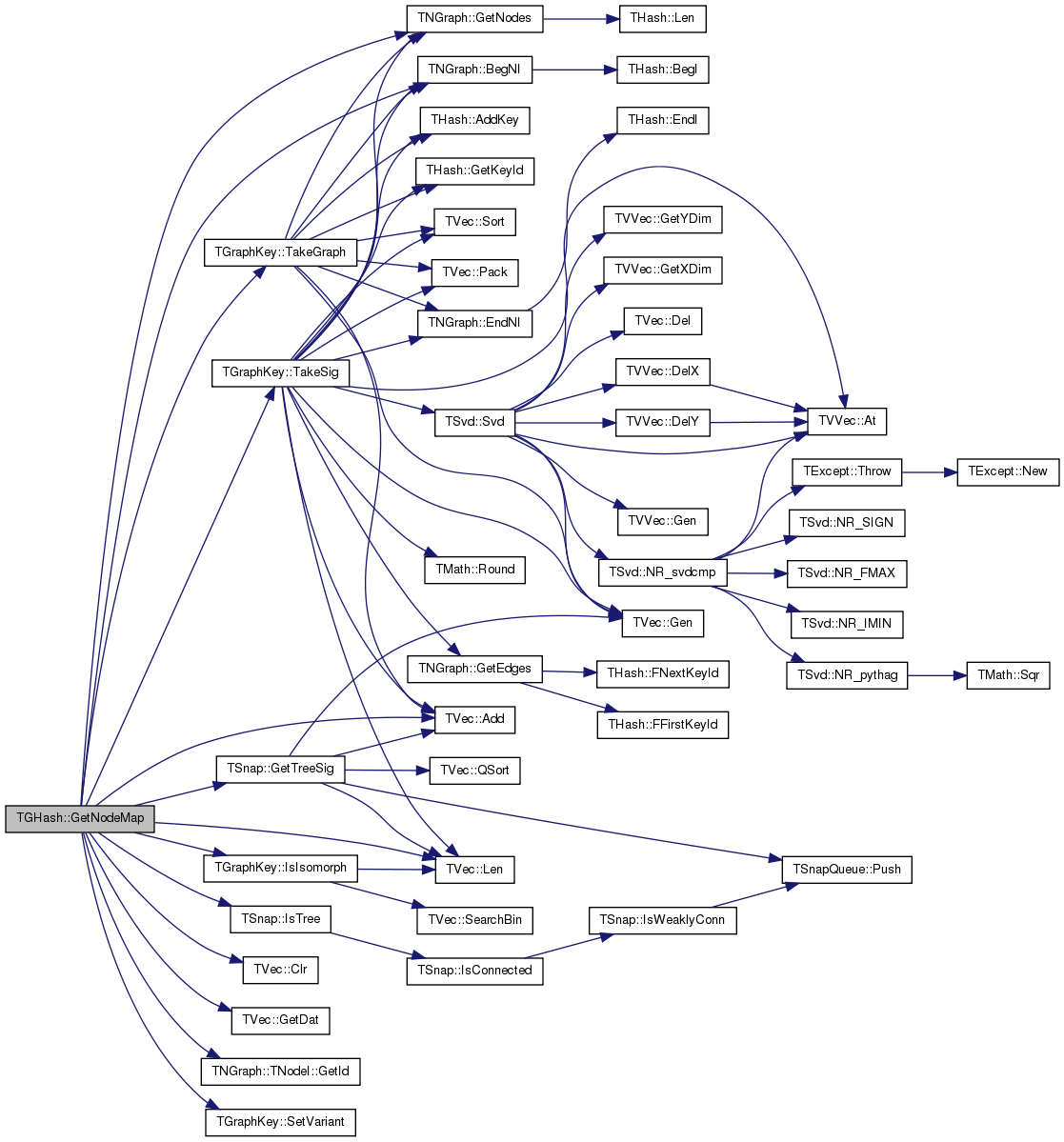template<class TDat>
 int TGHash< TDat >::GetPorts ( ) const
inline

Returns the number of ports in the hash table.

Definition at line 160 of file ghash.h.

160 { return GraphH.GetPorts(); }
int GetPorts() const
Definition: hash.h:229
THash< TGraphKey, TDat > GraphH
Definition: ghash.h:111
template<class TDat>
 bool TGHash< TDat >::HashTrees ( ) const
inline

Returns whether the hash table only hashes trees and not arbitrary directed graphs.

Definition at line 146 of file ghash.h.

146 { return HashOnlyTrees; }
TBool HashOnlyTrees
Definition: ghash.h:110
template<class TDat >
 void TGHash< TDat >::InitPermutations ( )
private

Definition at line 287 of file ghash.h.

Referenced by TGHash< TDat >::TGHash().

287  {
288  GSzToPermH.Clr();
289  for (int nodes = 2; nodes <= MxIsoCheck; nodes++) {
290  TVec<TIntV> NodePermutationV;
291  TIntV NodeIdV(nodes, 0);
292  for (int i = 0; i < nodes; i++) NodeIdV.Add(i);
293  NodeIdV.Pack();
295  while (NodeIdV.NextPerm()) {
297  }
298  NodePermutationV.Pack();
300  }
301 }
THash< TInt, TVec< TIntV > > GSzToPermH
Definition: ghash.h:109
TInt MxIsoCheck
Definition: ghash.h:107
void Pack()
Reduces vector capacity (frees memory) to match its size.
Definition: ds.h:1057
Adds a new element at the end of the vector, after its current last element.
Definition: ds.h:602

Here is the call graph for this function: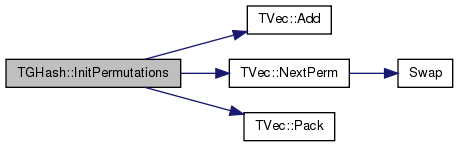Here is the caller graph for this function: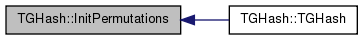template<class TDat>
 bool TGHash< TDat >::IsAutoSize ( ) const
inline

Tests whether the hash table automatically adjusts the number of ports based on the number of keys.

Definition at line 162 of file ghash.h.

162 { return GraphH.IsAutoSize(); }
THash< TGraphKey, TDat > GraphH
Definition: ghash.h:111
bool IsAutoSize() const
Definition: hash.h:230
template<class TDat >
 int TGHash< TDat >::IsGetKeyId ( const PNGraph & Graph ) const
private

Definition at line 370 of file ghash.h.

Referenced by TGHash< TUInt64 >::GetKeyId(), and TGHash< TUInt64 >::IsKey().

370  {
371  TGraphKey GKey;
372  return IsGetKeyId(Graph, GKey);
373 }
Small Directed Graphs.
Definition: ghash.h:7
int IsGetKeyId(const PNGraph &Graph) const
Definition: ghash.h:370

Here is the caller graph for this function:template<class TDat >
 int TGHash< TDat >::IsGetKeyId ( const PNGraph & Graph, TGraphKey & GKey ) const
private

Definition at line 376 of file ghash.h.

376  {
377  if (HashOnlyTrees) {
378  // For trees we perform exact isomorshism test based on graph signatures
379  int RootNId; IAssert(TSnap::IsTree(Graph, RootNId));
380  TIntV TreeSig; TSnap::GetTreeSig(Graph, RootNId, TreeSig);
381  GKey = TGraphKey(TreeSig);
382  const int KeyId = GraphH.GetKeyId(GKey);
383  return KeyId;
384  } else {
385  // For small graphs of less than MxIsoCheck nodes we perform brute force isomorphism checking
386  GKey.TakeSig(Graph, MxIsoCheck+1, MxSvdGraph);
387  const int Nodes = GKey.GetNodes();
388  if (Nodes > 2 && Nodes <= MxIsoCheck) {
389  GKey.TakeGraph(Graph);
390  for (int variant = 1; ; variant++) {
391  GKey.SetVariant(variant);
392  int KeyId = GraphH.GetKeyId(GKey); // Is there a graph of the same signature and same VariantId
393  if (KeyId == -1) { return -1; }
394  if (TGraphKey::IsIsomorph(GKey, GraphH.GetKey(KeyId), GSzToPermH.GetDat(Nodes))) { return KeyId; } // perform brute force isomorphism check
395  }
396  } else {
397  // For all other graphs we perform approximate graph isomorphism checking
398  const int KeyId = GraphH.GetKeyId(GKey);
399  return KeyId;
400  }
401  }
402  Fail;
403  return -1;
404 }
void TakeSig(const PNGraph &Graph, const int &MnSvdGraph, const int &MxSvdGraph)
Creates a signature for a given directed graph.
Definition: ghash.cpp:94
#define IAssert(Cond)
Definition: bd.h:262
TBool HashOnlyTrees
Definition: ghash.h:110
#define Fail
Definition: bd.h:238
THash< TInt, TVec< TIntV > > GSzToPermH
Definition: ghash.h:109
static bool IsIsomorph(const TGraphKey &Key1, const TGraphKey &Key2, const TIntV &NodeIdMap)
Checks whether directed graph Key1 is isomorphic to the directed graph Key2 under node Id permutation...
Definition: ghash.cpp:186
void GetTreeSig(const PGraph &Graph, const int &RootNId, TIntV &Sig)
Definition: alg.h:484
bool IsTree(const PGraph &Graph, int &RootNIdX)
Definition: alg.h:460
TInt MxIsoCheck
Definition: ghash.h:107
TInt MxSvdGraph
Definition: ghash.h:108
void TakeGraph(const PNGraph &Graph)
Creates a key from a given directed graph.
Definition: ghash.cpp:58
int GetNodes() const
Returns the number of nodes in the graph.
Definition: ghash.h:31
int GetKeyId(const TKey &Key) const
Definition: hash.h:466
Small Directed Graphs.
Definition: ghash.h:7
THash< TGraphKey, TDat > GraphH
Definition: ghash.h:111
void SetVariant(const int &Variant)
Sets the Variant Id of a given graph.
Definition: ghash.h:47
const TKey & GetKey(const int &KeyId) const
Definition: hash.h:252

Here is the call graph for this function: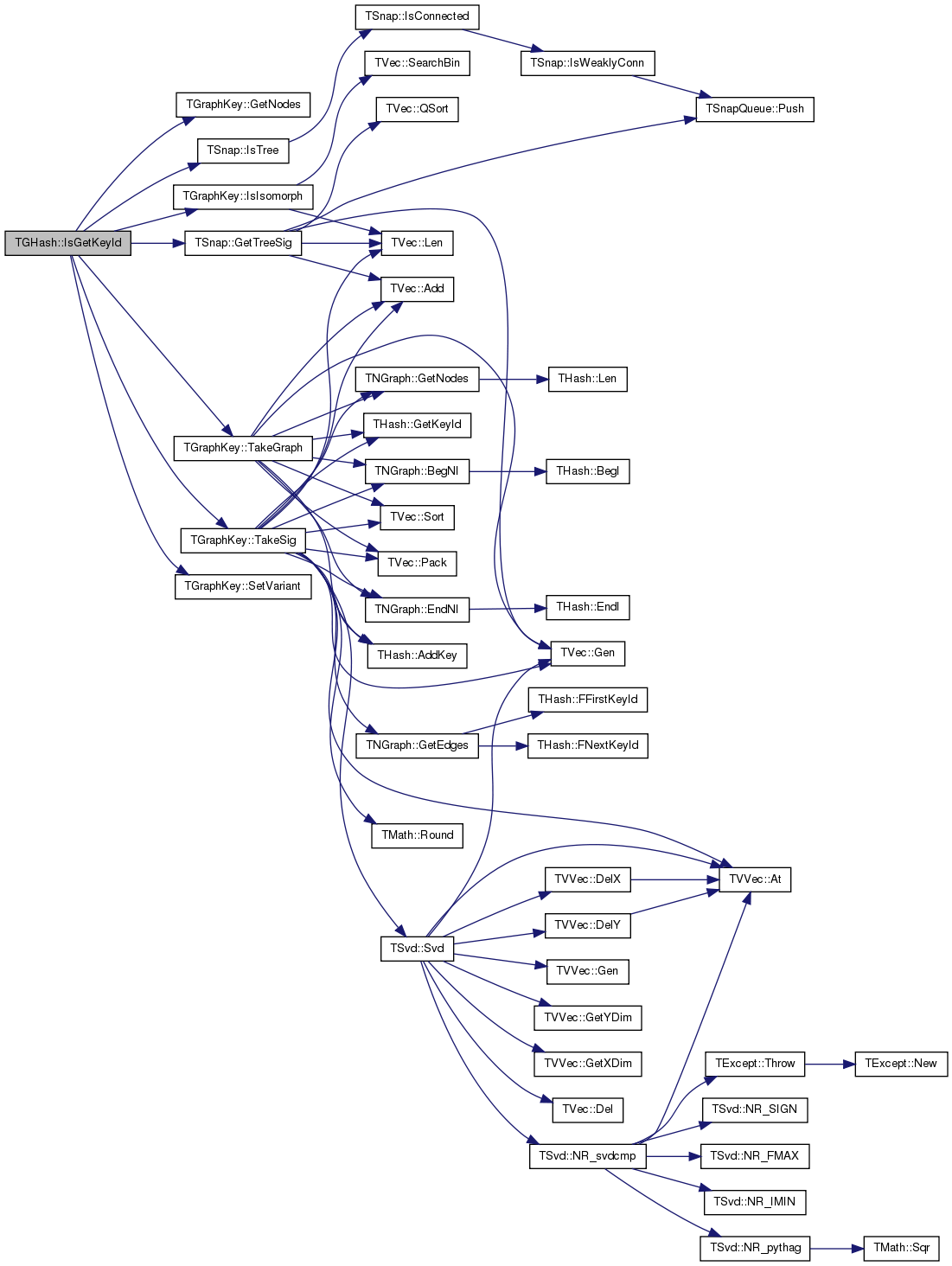template<class TDat>
 int TGHash< TDat >::IsGetKeyId ( const PNGraph & Graph, TGraphKey & GKey, TIntPrV & NodeMap ) const
private
template<class TDat>
 bool TGHash< TDat >::IsKey ( const PNGraph & Graph ) const
inline

Test whether Graph is an existing key in the hash table.

Definition at line 184 of file ghash.h.

Referenced by TDGHashGraphCounter::operator()().

184 { int k=IsGetKeyId(Graph); return k!=-1; }
int IsGetKeyId(const PNGraph &Graph) const
Definition: ghash.h:370

Here is the caller graph for this function: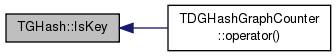template<class TDat>
 bool TGHash< TDat >::IsKey ( const TGraphKey & Key ) const
inline

Tests whether a given Key exists in the hash table.

Definition at line 205 of file ghash.h.

205 { return GraphH.IsKey(Key); }
THash< TGraphKey, TDat > GraphH
Definition: ghash.h:111
bool IsKey(const TKey &Key) const
Definition: hash.h:258
template<class TDat>
 bool TGHash< TDat >::IsKey ( const TGraphKey & Key, int & KeyId ) const
inline

Tests whether a given Key exists in the hash table.

Definition at line 207 of file ghash.h.

207 { return GraphH.IsKey(Key, KeyId); }
THash< TGraphKey, TDat > GraphH
Definition: ghash.h:111
bool IsKey(const TKey &Key) const
Definition: hash.h:258
template<class TDat>
 bool TGHash< TDat >::IsKeyGetDat ( const TGraphKey & Key, TDat & Dat ) const
inline

Test whether Key exists and sets its data to Dat.

Definition at line 230 of file ghash.h.

230 { return GraphH.IsKeyGetDat(Key, Dat); }
bool IsKeyGetDat(const TKey &Key, TDat &Dat) const
Definition: hash.h:274
THash< TGraphKey, TDat > GraphH
Definition: ghash.h:111
template<class TDat>
 bool TGHash< TDat >::IsKeyId ( const int & KeyId ) const
inline

Tests whether there exists a key at given position index KeyId.

Definition at line 209 of file ghash.h.

209 { return GraphH.IsKeyId(KeyId); }
bool IsKeyId(const int &KeyId) const
Definition: hash.h:260
THash< TGraphKey, TDat > GraphH
Definition: ghash.h:111
template<class TDat>
 bool TGHash< TDat >::IsKeyIdEqKeyN ( ) const
inline

Tests whether there are any unused slots in the hash table.

Slots get freed after removing keys from the table.

Definition at line 168 of file ghash.h.

168 { return GraphH.IsKeyIdEqKeyN(); }
bool IsKeyIdEqKeyN() const
Definition: hash.h:233
THash< TGraphKey, TDat > GraphH
Definition: ghash.h:111
template<class TDat>
 int TGHash< TDat >::Len ( ) const
inline

Returns the number of keys in the hash table.

Definition at line 158 of file ghash.h.

158 { return GraphH.Len(); }
THash< TGraphKey, TDat > GraphH
Definition: ghash.h:111
int Len() const
Definition: hash.h:228
template<class TDat>
 const TDat& TGHash< TDat >::operator() ( const TGraphKey & Key ) const
inline

Accesses the data of graph-key Key.

Definition at line 135 of file ghash.h.

135 { return GraphH.GetDat(Key); }
const TDat & GetDat(const TKey &Key) const
Definition: hash.h:262
THash< TGraphKey, TDat > GraphH
Definition: ghash.h:111
template<class TDat>
 TDat& TGHash< TDat >::operator() ( const TGraphKey & Key )
inline

Accesses the data of graph-key Key.

Definition at line 137 of file ghash.h.

137 { return GraphH.GetDat(Key); }
const TDat & GetDat(const TKey &Key) const
Definition: hash.h:262
THash< TGraphKey, TDat > GraphH
Definition: ghash.h:111
template<class TDat>
 const TDat& TGHash< TDat >::operator[] ( const int & KeyId ) const
inline

Accesses the data at hash table position index KeyId.

Definition at line 131 of file ghash.h.

131 { return GraphH[KeyId]; }
THash< TGraphKey, TDat > GraphH
Definition: ghash.h:111
template<class TDat>
 TDat& TGHash< TDat >::operator[] ( const int & KeyId )
inline

Accesses the data at hash table position index KeyId.

Definition at line 133 of file ghash.h.

133 { return GraphH[KeyId]; }
THash< TGraphKey, TDat > GraphH
Definition: ghash.h:111
template<class TDat>
 void TGHash< TDat >::Pack ( )
inline

Frees the unused memory by the hash table.

Definition at line 274 of file ghash.h.

274 { GraphH.Pack(); }
THash< TGraphKey, TDat > GraphH
Definition: ghash.h:111
void Pack()
Definition: hash.h:289
template<class TDat >
 void TGHash< TDat >::Save ( TSOut & SOut ) const

Definition at line 319 of file ghash.h.

319  {
320  MxIsoCheck.Save(SOut);
321  MxSvdGraph.Save(SOut);
322  HashOnlyTrees.Save(SOut);
323  GraphH.Save(SOut);
324 }
TBool HashOnlyTrees
Definition: ghash.h:110
void Save(TSOut &SOut) const
Definition: dt.h:1153
void Save(TSOut &SOut) const
Definition: hash.h:183
void Save(TSOut &SOut) const
Definition: dt.h:995
TInt MxIsoCheck
Definition: ghash.h:107
TInt MxSvdGraph
Definition: ghash.h:108
THash< TGraphKey, TDat > GraphH
Definition: ghash.h:111
template<class TDat >
 void TGHash< TDat >::SaveDetailTxt ( const TStr & OutFNm, const TStr & Desc, const TStr & DatColNm ) const

Saves all graphs stored in the hash table into a text file and include additional information.

Definition at line 523 of file ghash.h.

References TStr::CStr(), and TVec< TVal, TSizeTy >::Len().

523  {
524  TIntV KeyIdV; GetKeyIdByDat(KeyIdV, false);
525  FILE *F = fopen(OutFNm.CStr(), "wt");
526  fprintf(F, "Graph-Hash-Table\n");
527  fprintf(F, "%s\n", Desc.CStr());
528  fprintf(F, "%d graphs", KeyIdV.Len());
529  for (int i = 0; i < KeyIdV.Len(); i++) {
530  fprintf(F, "\n\n[%5d]\tRank: %d\n", KeyIdV[i](), i+1);
531  fprintf(F, "Dat: %s\n", GetDat(KeyIdV[i]).GetStr().CStr());
532  GetDatId(KeyIdV[i]).SaveTxt(F);
533  }
534  fclose(F);
535 }
TSizeTy Len() const
Returns the number of elements in the vector.
Definition: ds.h:575
const TDat & GetDat(const PNGraph &Graph) const
Returns the data associated with key Graph.
Definition: ghash.h:192
const TDat & GetDatId(const int &KeyId) const
Returns data at a given position index KeyId.
Definition: ghash.h:221
char * CStr()
Definition: dt.h:479
void GetKeyIdByDat(TIntV &KeyIdV, const bool &Asc=true) const
Returns a vector of KeyIds of hash table elements sorted by their data value.
Definition: ghash.h:454

Here is the call graph for this function:template<class TDat >
 void TGHash< TDat >::SaveTxt ( const TStr & OutFNm, const TStr & Desc, const TStr & DatColNm, const bool & SortByKeyVal = `true` ) const

Saves all graphs stored in the hash table into a text file.

Definition at line 505 of file ghash.h.

505  {
506  TIntV KeyIdV;
507  if (SortByKeyVal) GetKeyIdByDat(KeyIdV, false);
508  else GetKeyIdByGSz(KeyIdV, true);
509  FILE *F = fopen(OutFNm.CStr(), "wt");
510  fprintf(F, "Graph-Hash-Table");
511  fprintf(F, "%s\n", Desc.CStr());
512  fprintf(F, "%d graphs\n", KeyIdV.Len());
513  fprintf(F, "Rank\tKeyId\tNodes\tEdges\t%s\n", DatColNm.CStr());
514  for (int i = 0; i < KeyIdV.Len(); i++) {
515  const TGraphKey& Key = GetKey(KeyIdV[i]);
516  fprintf(F, "%d\t%d\t%d\t%d\t%s\n", i+1, KeyIdV[i](), Key.GetNodes(), Key.GetEdges(),
517  GetDatId(KeyIdV[i]).GetStr().CStr());
518  }
519  fclose(F);
520 }
int GetEdges() const
Returns the number of edges in the graph.
Definition: ghash.h:33
const TGraphKey & GetKey(const int &KeyId) const
Returns the GraphKey with position index KeyId.
Definition: ghash.h:199
void GetKeyIdByGSz(TIntV &KeyIdV, const bool &Asc=true) const
Returns a vector of KeyIds of hash table elements sorted by their graph size.
Definition: ghash.h:467
TSizeTy Len() const
Returns the number of elements in the vector.
Definition: ds.h:575
int GetNodes() const
Returns the number of nodes in the graph.
Definition: ghash.h:31
const TDat & GetDatId(const int &KeyId) const
Returns data at a given position index KeyId.
Definition: ghash.h:221
Small Directed Graphs.
Definition: ghash.h:7
char * CStr()
Definition: dt.h:479
void GetKeyIdByDat(TIntV &KeyIdV, const bool &Asc=true) const
Returns a vector of KeyIds of hash table elements sorted by their data value.
Definition: ghash.h:454

Here is the call graph for this function: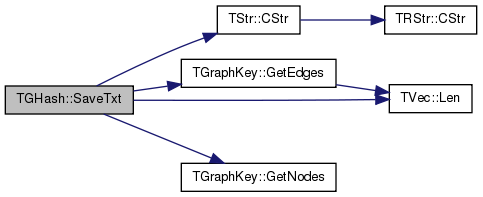## Member Data Documentation

template<class TDat>
 THash TGHash< TDat >::GraphH
private

Definition at line 111 of file ghash.h.

template<class TDat>
 THash > TGHash< TDat >::GSzToPermH
private

Definition at line 109 of file ghash.h.

template<class TDat>
 TBool TGHash< TDat >::HashOnlyTrees
private

Definition at line 110 of file ghash.h.

Referenced by TGHash< TUInt64 >::HashTrees(), and TGHash< TDat >::TGHash().

template<class TDat>
 TInt TGHash< TDat >::MxIsoCheck
private

Definition at line 107 of file ghash.h.

template<class TDat>
 TInt TGHash< TDat >::MxSvdGraph
private

Definition at line 108 of file ghash.h.

The documentation for this class was generated from the following file: